# ISEE Upper Level Quantitative : How to multiply exponents

## Example Questions

← Previous 1

### Example Question #1 : How To Multiply Exponents

Simplify: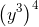The correct answer is not among the other choices.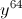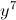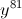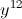Explanation:

Apply the power of a power property: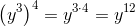### Example Question #2 : How To Multiply Exponents

Simplify the expression: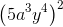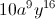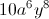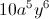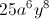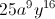Explanation:

Apply the power of a product rule, then apply the power of a power rule: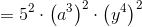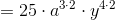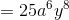### Example Question #2 : How To Multiply Exponents

Which of the following expressions is equal to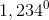?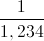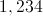The expression is undefined.Explanation:

Any nonzero number raised to the power of 0 is equal to 1.

### Example Question #3 : How To Multiply Exponents

Which quantity is greater?

(a)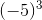(b)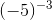It is impossible to tell from the information given.

(a) and (b) are equal.

(a) is greater.

(b) is greater.

(b) is greater.

Explanation:

(a)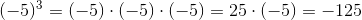(b)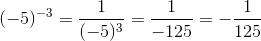(b) is the greater quantity.

### Example Question #461 : Isee Upper Level (Grades 9 12) Quantitative Reasoningis positive.

Which is the greater quantity?

(a)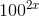(b)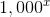(a) is greater

(b) is greater

(a) and (b) are equal

It is impossible to tell from the information given

(a) is greater

Explanation:

Use the power of a power property:

(a)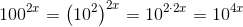(b)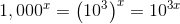Since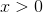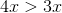. Subsequently,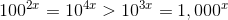,

making (a) greater

### Example Question #4 : How To Multiply Exponents

Which is the greater quantity?

(a)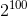(b)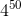(b) is greater

(a) is greater

It is impossible to tell from the information given

(a) and (b) are equal

(a) and (b) are equal

Explanation: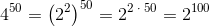The two quantities are equal.

### Example Question #1 : Exponential Operations

Two quantities are given - one in Column A and the other in Column B. Compare the quantities in the two columns.

Assume, in both columns, that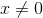.

Column A                    Column B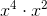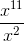The quantity in Column B is greater.

The quantity in Column A is greater.

The quantities in both columns are equal.

There is no way to determine the relationship between the columns.

There is no way to determine the relationship between the columns.

Explanation:

Column A gives simplifies to give us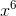, and Column B simplifies to give us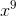. At first glance, Column B is greater, as it would be for all answers greater than 1. However, if, the two columns are equal. Furthermore, ifis negative, or a fraction, Column A is greater. Thus, since we could arrive at all three answers by using different numbers, we cannot determine the answer conclusively.

### Example Question #8 : How To Multiply Exponents

Which of the following expressions is equivalent to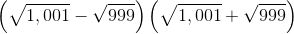?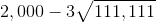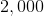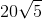Explanation:

Use the difference of squares pattern as follows: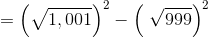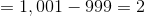### Example Question #5 : How To Multiply Exponents

Column A                  Column B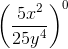The quantity in Column A is greater.

The quantities in both columns are equal.

The quantity in Column B is greater.

No relationship between the columns can be determined.

The quantity in Column A is greater.

Explanation:

Anything raised to zero is equal to 1. Therefore, Column A has to be greater because 1 is greater than 0.

### Example Question #6 : Exponential Operations

44,000,000 can be written in scientific notation as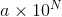for some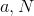.

Which is the greater quantity?

(A)(B) 8

(B) is greater

It is impossible to determine which is greater from the information given

(A) is greater

(A) and (B) are equal

(B) is greater

Explanation:

To write 44,000,000 in scientifc notation, write the implied decimal point after the final "0", then move it left until it is after the first nonzero digit (the first "4").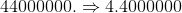This requires a displacement of seven places, so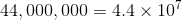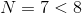, and (B) is greater.

← Previous 1